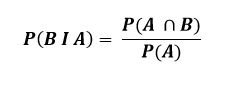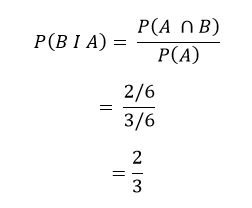Select Page

# Independent and Dependent Events

The events A and B are said to be independent if the occurrence or non-occurrence of event A does not affect the probability of occurrence of B. This means that irrespective whether event A has occurred or not, the probability of B is going to be the same. If the events A and B are not independent, they are said to be dependent.

For example, if we toss two coins, the occurrence and non-occurrence of a head one coin does not in any way effect the occurrence of a head on the other coin. Thus the two coins are independent. Similarly, suppose event A is the drawing of an ace from the pack of 52 cards and event B is throwing a total of a 7 with two dice. The event A and B are independent because drawing of a card does not affect the throwing of a total of 7.

On the other hand, if event A is drawing an ace on the first draw from the pack of cards and event B is drawing an ace on the second draw, it is obvious that the occurrence and non-occurrence of the first event does affect the probability of second event. We say that these two events are not independent; they are dependent events.

In the game of chance, such as tossing a coin or rolling a die, it is always assumed that successive throws are independent events if the coin or the die is fair. It is important to remember that event A is independent of event B when B is independent of A. because of this mutual independence, it is right to say that A and B are independent without specifying which is independent of the other.

# Conditional Probability

The probability of the occurrence of an event A when it is known that some other event B has already occurred is called conditional probability of A given that the event B has already occurred and is denoted by P(A I B) is usually as “the probability that A occurs given that B has already occurred” or simply “the probability of A given B”

To illustrate the concept of conditional probability let us consider an example. Suppose a die is rolled. The sample space of this experiment is S = {1,2, 3, 4, 5, 6}. The probability of getting a ‘6’ (event A) is 1/6. i.e. P(A) = 1/6. Suppose we are told that one particular through of a die, the outcome is an even number (event B). B = {2, 4, 6} and the probability of getting a ‘6’ in this reduced sample space is 1/3. This is the conditional probability of occurrence of event A (getting a ‘6’) given that B (getting an even number) has occurred, i.e. P(A I B) = 1/3.
Mathematically, if events A and B belongs to the sample space S and if P(B) ≠ 0, then the conditional probability of A given that B has occurred, denoted by P(A I B), is defined asIf we interchange the roles of A and B and if P(A) ), then the conditional probability of B given that A has occurred, denoted by P(B I A), is given by## Conditional Probability Example

A fair die is rolled once. Given that the outcome is even , what is the probability of getting a number greater than 3?

Let us first define the following sets:

• S = {1, 2, 3, 4, 5, 6} (sample space)
• A = {2, 4, 6} (set of even numbers)
• B = {4, 5, 6} (set of numbers greater than 3)
• A  B = {4, 6} (set of even numbers which are greater than 3)

Since a die is fair, we assign a probability of 1/6 to each sample point. Thus P(A) = 3/6 and P(A  B) = 2/6. Using the definition of conditional probability, we can now determine the probability of getting a number greater than 3  given that the outcome is even asThis result can be verified by the fact that out of three even outcomes {2, 4, 6} only two are greater than 3.

To understand the complete topic of probability, read the following lectures below.

Probability Definition

Sample Space, Events and Experiment

Shares